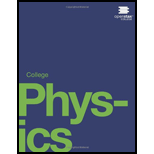# During heavy lifting, a disk between spinal vertebrae is subjected to a 5000-N compressional force. (a) What pressure is created, assuming that the disk has a uniform circular cross section 2.00 cm in radius? (b) What deformation is produced if the disk is 0.800 cm thick and has a Young's modulus of 1.5 × 10 9 N / m 2 ?### College Physics

1st Edition
Paul Peter Urone + 1 other
Publisher: OpenStax College
ISBN: 9781938168000### College Physics

1st Edition
Paul Peter Urone + 1 other
Publisher: OpenStax College
ISBN: 9781938168000

#### Solutions

Chapter
Section
Chapter 11, Problem 77PE
Textbook Problem

## During heavy lifting, a disk between spinal vertebrae is subjected to a 5000-N compressional force. (a) What pressure is created, assuming that the disk has a uniform circular cross section 2.00 cm in radius? (b) What deformation is produced if the disk is 0.800 cm thick and has a Young's modulus of 1.5 × 10 9 N / m 2 ?

Expert Solution
To determine

(a)

The pressure created when the disk between the spinal vertebrae is compressed.

### Explanation of Solution

Given:

The compressional force

F=5000 N

r=2.00 cm=2.00×102m

Formula used:

The pressure created when the disk is compressed is given by

P=FA

Here, A is the area of the disk, given by,

A=πr2

Calculation:

Calculate the area of the disk.

A=πr2=(3.14)(2.00× 10 2m)2=1

Expert Solution
To determine

(b)

The deformation produced in the disk.

### Want to see the full answer?

Check out a sample textbook solution.See solution

### Want to see this answer and more?

Bartleby provides explanations to thousands of textbook problems written by our experts, many with advanced degrees!

See solution